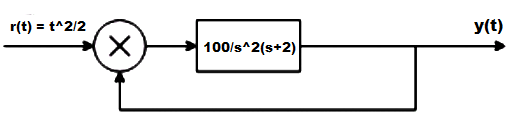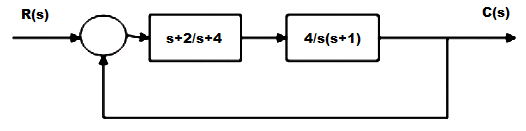# Steady-state Errors & Error Constants

Steady-state Errors & Error Constants

1. The steady state error for a unity feedback system for the input r(t) = Rt^2/2 to the system G(s) = K(s+2)/s(s3+7s2+12s) is

a) 0
b) 6R/K
c) ∞
d) 3R/K

Explanation: Ka = 2K/12 = K/6
Ess = 6R/K.

2. A control system is operated with a parabolic input as shown belowIf Ka is static error constant of the system, then its dynamic error constant K2 is

a) 0.01
b) 0.02
c) 0.03
d) 0.04

Explanation: Ka = 100/(s+2)
K2 = 1/50 = 0.02.

3. Find the velocity error constant of the system given below :a) 0
b) 2
c) 4
d) ∞

Explanation: Comparing with the characteristic equation the values of G and w are calculated as G = 1 and w = 4 and hence the system is critically damped.

4. Consider the unity feedback system with open loop transfer function the minimum value of the steady state error to a ramp input r(t) = 6tu(t) is OLTF = K/s(s+1)(s+2)

a) 1
b) 2
c) 3
d) 4

Explanation: Routh-Hurwitz criterion is used to calculate the stability of the system by checking the sign changes of the first row and from Routh-Hurwitz criterion maximum value of K = 6 ess = A/Kv for type-1 system and A = 6then for Kv = K/2, ess = 2.

5. A ramp input applied to a unity feedback system results in 5% steady state error. The type number and zero frequency gain of the system are respectively

a) 1 and 20
b) 0 and 20
c) 0 and 1/20
d) 1 and 1/20

Explanation: Steady state error is the error calculated between the final output and desired output and for the good control system the error must be less and this steady state error is inversely proportional to gain.

6. A particular control system yielded a steady state error of 0.20 for unit step input. A unit integrator is cascaded to this system and unit ramp input is applied to this modified system. What is the value of steady-state error for this modified system?

a) 0.10
b) 0.15
c) 0.20
d) 0.25

Explanation: The integrator is similar to the phase lag systems and it is used to reduce or eliminate the steady state error and when it is cascaded with the ramp input hence the acceleration error constant is calculated which is equal to 0.25.

7. The error constants described are the ability to reduce the steady state errors.

a) True
b) False

Explanation: As the type of the system becomes higher more steady state errors are eliminated.

8. Systems of type higher than 2 are not employed in practice.

a) True
b) False

Explanation: These are more difficult to stabilize and dynamic errors are much larger.

9. Steady state refers to

a) Error at the steady state
b) Error at the transient state
c) Error at both state
d) Precision

Explanation: Steady state errors are the change in the output at the steady state with respect to the change in the input.

10. The disadvantages of the error constants are:

a) They do not give the information of the steady state error when the inputs are other than the three basic types
b) Error constant fail to indicate the exact manner in which the error function change with time.
c) They do not give information of the steady state error and fail to indicate the exact manner in which the error function change with time
d) They give information of the steady state error